Drive_Index
Gears Gearboxes

Epicyclic Gears

Introduction

An epicylic gear is a planetary gear arrangement consisting of one or more planet(epicyclic) gears (P) meshed and rotating round a central sun gear (S).   The planet gears are also meshed and rotate within an internal ring gear (A).   The planet gears are fixed to a planet carrier-crank arm(L) designed to rotate on the same centre as the sun gear.  Only one planet-carrier /crank arm is used in a single epicyclic gear train.   This complicated arrangement (see below) has a number of modes of operation depending on which members are locked.  Epicyclic gears can be based on spur gears, helical gears, or bevel gears.

Epicyclic gearboxes are generally purchased as complete units from specialist suppliers.

Design Features
 Co-axial drive and driven shafts Compact drive Low moment of Inertia Torque transmitted up to 10,000 Nm Drive speed up to 15,000 RPM Efficient Quiet in operation

Epicyclic Gearboxes (Planetary gearboxes)have the following features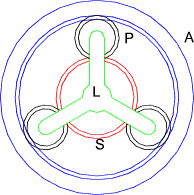Typical Epicyclic Gear Arrangement

There are three configurations possible with this epicylic gear

Star where the planet carrier arm is fixed and the sun and annulus ring gear rotate.

�Fixed frame assembly
�Input and output shaft rotate in opposite directions
�Ratios up to 10:1 (approx)-- (Reduction or increase depending on drive direction.)

Planetary where the annulus ring gear is the fixed component and the sun gear and planet carrier arm rotate

�Fixed annulus system
�Input and output shaft rotate in same directions
�Ratios up to 12:1 (approx)-- (Reduction or increase depending on drive direction.)

Solar where the sun gear is the fixed component and the annulus ring gear and planet carrier arm rotate.

�Fixed sunwheel
�Input and output shafts rotate is same direction
�Ratios up to 2:1 (approx) -- (Reduction or increase depending on drive direction.)

Terminology

The terminology used below is
NS, NA, NP = number of teeth on the sun wheel, the ring gear, and the plant gears respectively.
RS, RA, RL RP = rotation (revs) of the the sun wheel, the ring gear, the planatory arm, and the plant gears respectively.
ω S ,ω A ,ω L,ω P = angular velocity of the sun wheel, the ring gear, the planatory arm, and the plant gears respectively.

If the ratio of rotation of the follower member (RA) has a ratio of R relative to the driving member (RB) rotation i.e. RA = R. RB. Then RB = RA /R

Calculation of Ratios

Example 1.----Planetary Arrangement.

The driver is the planetary arm ( L ).
The driven member is the Sun (S).
The Ring Gear is fixed. (A)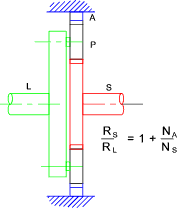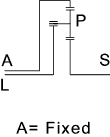Note NA = NS + 2. NP

 Action Rotation (CW= +ve) from action....................Nx = number of teeth L A P S Turn whole gear thro 1 rev Clock Wise 1 1 1 1 Fix arm L and rotate A back CCW 1 rev 0 -1 -NA /NP +NA / NS Add the two motions above 1 0 1 - ( NA / NP) 1 + ( NA / NS)

Summary : Rotation of the planet shaft(L) 1 rev CW results in the rotation of the shaft (S) 1 + ( NA / NS) revs (CW).
Ratio = RS = RL ( 1 + ( NA / NS)
ratio r1 = RS / RL = ( 1 + ( NA / NS)

 Example 1a.. Arrangement as above The driver is the Sun ( S ). The driven member is the planetary arm (L). The Ring Gear is fixed. (A) The analysis is as above resulting in a ratio of RS = RL ( 1 + ( NA / NS) if the driver is the sun wheel The ratio for this inverse arrangement is RL = RS / ( 1 + ( NA / NS) ratio r1a = RL / RS = 1 / ( 1 + ( NA / NS)

Example 2..----Star Arrangement

The driver is the Sun ( S ).
The driven member is the Ring (A).
The planetary arm is fixed. (L)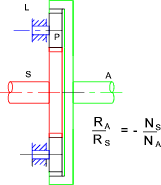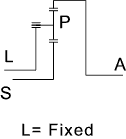Note NA = NS + 2. NP

 Action Rotation (CW= +ve) from action....................Nx = number of teeth S L P A Fix L and rotate Sun 1 rev CW 1 0 - NS / NP - NS / NA

Summary : Rotation of the Sun shaft(S) 1 rev CW results in the rotation of the shaft (A) NS / NA revs (CCW).
Ratio = RA = RS( - NS / NA )
ratio r2 = RA / RS = - ( NS / NA)

 Example 2a.. Arrangement as above The driver is the ring gear ( A ). The follower is the Sun (S). The the planetary arm (L) is fixed. ) The analysis is as above resulting in a ratio of Ratio RA = RS( - NS / NA ) if the driver is the ring wheel The ratios for this inverse arrangement is RS = RA( - NA / NS )) ratio r2a = RS / RA = - ( NA / NS)

Example 3.----Solar Arrangement..

The driver is the planetary Arm ( L ).
The follower is the annulus ring (A)
The the sun gear (S) is fixed.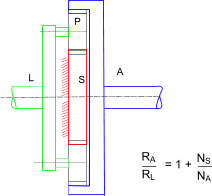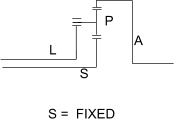Note NA = NS + 2. NP

 Action Rotation (CW= +ve) from action....................Nx = number of teeth L A P S Turn whole gear thro 1 rev Clock Wise 1 1 1 1 Fix arm L and rotate S back CCW 1 rev 0 NS /NA NS /NP -1 Add the two motions above 1 1 + ( NS / NA) 1 + ( NS / NP) 0

Summary : Rotation of the Planet arm L 1 rev CW results in the rotation of the Ring gear (A) 1 + ( NS / NA)revs CW.
Ratio = RA = RL ( 1 + ( NS / NA)
ratio r3 = RA / RL = ( 1 + ( NS / NA)

 Example 3a.. Arrangement as above The driver is the annulus ring gear (A) The follower is the planetary arm (L). The sun ( S )is fixed. The analysis is as above resulting in a ratio of Ratio RA = RL.( 1 + ( NS / NA)) if the driver is the sun wheel and the follower is the planetary arm the resulting ratio is RL = RA / ( 1 + ( NS / NA) ratio r3a = RL / RA = 1 / ( 1 + ( NS / NA)

Example 4..

The driver is the planetary Arm ( L ).
The follower is the sun (S).
The the Ring gear (A) is fixed.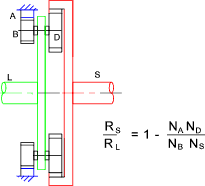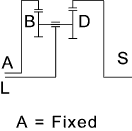Note NS = NA - NB + ND

 Action Rotation (CW= +ve) from action....................Nx = number of teeth L A B-D S Turn whole gear through 1 rev CW 1 1 1 1 Fix L and rotate A back CCW 1 rev 0 -1 - NA / NB - ( NA / NB).( N D / N S) Add the two motions above 1 0 1 -( NA / NB) 1 - ( NA . ND)/( N B . N S)

Summary : Rotation of the Shaft L 1 rev CW results in the rotation of the shaft (S) 1 - ( NA . ND)/( N B . N S) revs (CW).
Ratio = RS = RL [1 - ( NA . ND)/( N B. N S)]

ratio r4 = RS / RL = [1 - ( NA . ND)/( N B. N S)]

 Example 4a.. Arrangement as above The driver is the sun ( S ). The follower is the planetary arm (L). The the Ring gear (A) is fixed. ) The analysis is as above resulting in a ratio of Ratio RS = RL[1 - ( NA . ND)/( N B. N S)] if the driver is the sun wheel and the follower is the planetary arm the resulting ratio is RL = RS / [1 - ( NA . ND)/( N B. N S)] ratio r4a = RL / RS = 1 / [1 - ( NA . ND)/( N B. N S)]

Example 5..

The driver is the planetary Arm ( L ).
The follower is the sun (S).
The the Ring gear (A) is fixed. )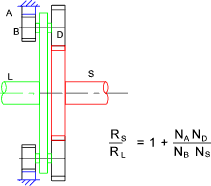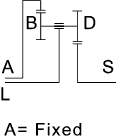Note NS = NA - NB - ND

 Action Rotation (CW= +ve) from action....................Nx = number of teeth L A B-D S Turn whole gear through 1 rev CW 1 1 1 1 Fix L and rotate A back CCW 1 rev 0 -1 - NA / NB + ( NA / NB).( N D / N S) Add the two motions above 1 0 1 -( NA / NB) 1 + ( NA . ND)/( N B . N S)

Summary : Rotation of the shaft L 1 rev CW results in the rotation of the shaft (S) 1 + ( NA . ND)/( N B . N S) revs (CW).
Ratio : RS = RL [1 + ( NA . ND)/( N B . N S)]
ratio r5 = RS / RL = [1 + ( NA . ND)/( N B. N S)]

 Example 5a.. Arrangement as above The driver is the sun ( S ). The follower is the planetary arm (L). The the Ring gear (A) is fixed. ) The analysis is as above resulting in a ratio of Ratio RS = RL[1 + ( NA . ND)/( N B. N S)] if the driver is the sun wheel and the follower is the planetary arm the resulting ratio is RL = RS / [1 + ( NA . ND)/( N B. N S)] ratio r5a = RL / RS = 1 / [1 + ( NA . ND)/( N B. N S)]

Example 6..

The driver is the planetary Arm ( L ).
The follower is the sun (S).
The the Ring gear (A) is fixed.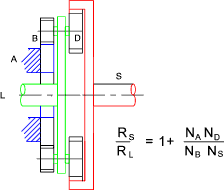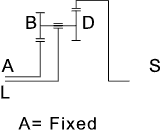Note NS = NA + NB + ND

 Action Rotation (CW= +ve) from action....................Nx = number of teeth L A B-D S Turn whole gear through 1 rev CW 1 1 1 1 Fix L and rotate A back CCW 1 rev 0 -1 + NA / NB + ( NA / NB).( N D / N S) Add the two motions above 1 0 1 + ( NA / NB) 1 + ( NA . ND)/( N B . N S)

Summary : Rotation of the shaft L - 1 rev CW results in the rotation of the shaft (S) 1 + ( NA . ND)/( N B . N S) revs (CW).
Ratio RS = RL [1 + ( NA . ND)/( N B . N S)]
ratio r6 = RS / RL = [1 + ( NA . ND)/( N B. N S)]

 Example 6a.. Arrangement as above The driver is the sun ( S ). The follower is the planetary arm (L). The the Ring gear (A) is fixed. ) The analysis is as above resulting in a ratio of Ratio RS = RL[1 + ( NA . ND)/( N B. N S)] if the driver is the sun wheel and the follower is the planetary arm the resulting ratio is RS = RL / [1 + ( NA . ND)/( N B. N S)] ratio r6a = RL / RS = 1 / [1 + ( NA . ND)/( N B. N S)]

Example 7..

The driver is the planetary Arm ( L ).
The follower is the sun (S).
The the Ring gear (A) is fixed.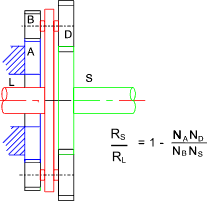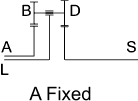Note NS = NA + NB - ND

 Action Rotation (CW= +ve) from action-...................... Nx = number of teeth L A B-D S Turn whole gear through 1 rev CW 1 1 1 1 Fix L and rotate A back CCW 1 rev 0 -1 + NA / NB - ( NA / NB).( N D / N S) Add the two motions above 1 0 1 + ( NA / NB) 1 - ( NA . ND)/( N B . N S)

Summary : Rotation of the shaft L 1 rev CW results in the rotation of the shaft (S) 1 - ( NA . ND)/( N B . N S) revs (CW).
Ratio 1: [1 - ( NA . ND)/( N B . N S)]
ratio r7 = RS / RL = [1 - ( NA . ND)/( N B. N S)]

 Example 7a.. Arrangement as above The driver is the sun ( S ). The follower is the planetary arm (L). The the Ring gear (A) is fixed. ) The analysis is as above resulting in a ratio of Ratio RS = RL[1 - ( NA . ND)/( N B. N S)] if the driver is the sun wheel and the follower is the planetary arm the resulting ratio is RL = RS / [1 - ( NA . ND)/( N B. N S)] ratio r7a = RL / RS = 1 / [1 - ( NA . ND)/( N B. N S)]

Epi-cyclic gear Train Variations

The figure below shows the range of possible epicylclic gear arrangements..   Those in section I & III are classed as simple arrangements because the planet gears mesh with both sun gears.   Those in sections II & IV are classed as complex trains because the planet gears partially match with each other and partially mesh with the sun gears.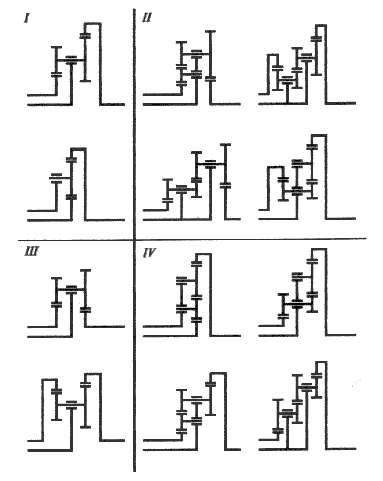Calculation of epicyclic Gear Ratio using Equations

1) First calculate the ratio of the gears with the planet carrier fixed..

r f = (±) Product of Driving Gear Teeth /Product of Driven Gear Teeth

Note: when two external gears are in contact there is a sign change (change of direction) when an internal gear meshes with an external gear both gears rotate in the same direction and there is no change in direction..

2) Designate and input gear (x) and an output gear (y).
The speed of the input gear relative to the carrier arm = ω x - ω L
The speed of the output gear relative to the carrier arm = ω y - ω L

3) The train value with the carrier fixed =

r f = ( ω y - ω L ) / ( ω x - ω L )

This relationship is used to solve the planetary gear train ratios.
Using this method for the examples above

Example 1.

1) r f = (NA /NP ).( - NP /NS )= - NA /NS
2) Select ω A as input speed = 0 and ω S as output speed (unknown) .
3) r f = ( ω S - ω L ) / ( ω A - ω L )   Therefore    - r f.ω L = ( ω S - ω L )   Therefore    ω S/ω L = 1 - r f

ω S/ω L = 1 + NA /NS

Example 2.

1) r f = (-NS /NP ).( NP /NA )= - NS /NA
2) Select ω S as input speed and ω A as output speed .ω L =0
3) r f = ( ω A - ω L ) / ( ω S - ω L )   Therefore   ω A /ω S = r f

ω A/ω S = - NS /NA

Example 3.

1) r f = (-NS /NP ).( NP /NA )= - NS /NA
2) Select ω S as input speed and ω L as output speed .ω S =0
3) r f = ( ω A - ω L ) / ( ω S - ω L )   Therefore r f = 1 - ωA / ω L
resulting in ωA / ω L = NS /NA + 1.

ω A/ω L = 1 + NS /NA

Example 4.

1) r f = (NA /NB ).(ND /NS )= ( N A.N D) / (NB .NS )
2) Select ω A as input speed = 0 and ω S as output speed. (solve for ω S/ ω L)
3) r f = ( ω S - ω L ) / ( ω A - ω L )   Therefore   1 - r f = ω S /ω L

ω S/ω L = 1 - ( N A.N D) / (NB .NS )

Example 5.

1) r f = (NA /NB ).( - ND /NS )= - ( N A.N D) / (NB .NS )
2) Select ω A as input speed = 0 and ω S as output speed. (solve for ω S/ ω L)
3) r f = ( ω S - ω L ) / ( ω A - ω L )   Therefore   1 - r f = ω S /ω L

ω S/ω L = 1 + ( N A.N D) / (NB .NS )

Example 6.

1) r f = (- NA /NB ).( ND /NS )= - ( N A.N D) / (NB .NS )
2) Select ω A as input speed = 0 and ω S as output speed. (solve for ω S/ ω L)
3) r f = ( ω S - ω L ) / ( ω A - ω L )   Therefore   1 - r f = ω S /ω L

ω S/ω L = 1 + ( N A.N D) / (NB .NS )

Example 7.

1) r f = (- NA /NB ).(- ND /NS )= ( N A.N D) / (NB .NS )
2) Select ω A as input speed = 0 and ω S as output speed. (solve for ω S/ ω L)
3) r f = ( ω S - ω L ) / ( ω A - ω L )   Therefore   1 - r f = ω S /ω L

ω S/ω L = 1 - ( N A.N D) / (NB .NS )

Calculating ratios by using the instantaneous planet gear velocities

This method analyses the motion of one planet gear. The centre moves at the same velocity as the carrier , the pitch velocity at the teeth mating with ring is the same as that of the pitch velocity of the ring and the pitch velocity of the teeth in contact with the sun gear moves at the same velocity as the pitch velocity of the sun gear.

Looking for example at the Planetary arrangement the ring gear is fixed vA = 0 , the driver is the carrier with a speed vL = ω L RL
Note: RL = (RA+ RS ) /2
The velocity at the pitch line mating with the Sun wheel vS is clearly 2.vL.
From this relationship the gear ratios can easily be calculated as shown below.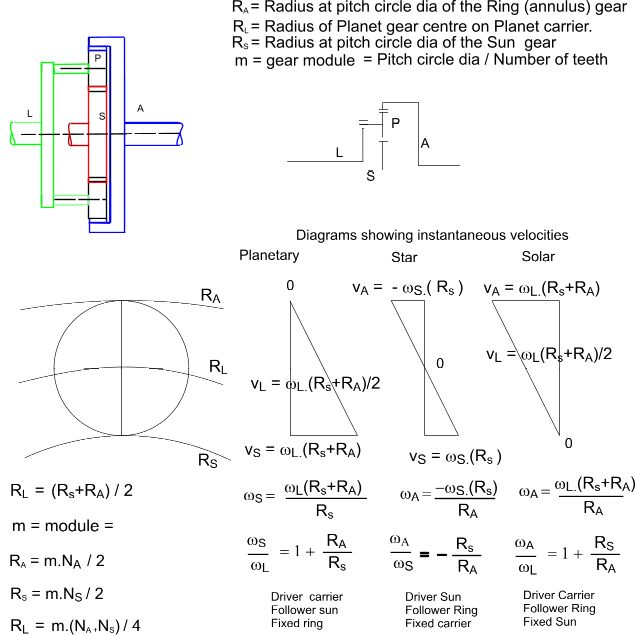Links to Epicyclic Gear Design DanNotes Epicyclic Gear Design ...Theoretical analysis include torque Epicyclic Gear Train Solution Techniques with Application to Tandem Bicycling...Informative Document Planetary gears...Informative paper Epi cyclic gear calculator...A basic calculator. ( terminolgy as on my my page ) Mitcalc.com -Gear calculations...Excel based programmes allowing detailed calculations. ExcelCalcs... Excellent facility for Engineers. Library includes epicyclic calculator based on Roymech.(Membership required).

Drive_Index
Gears Gearboxes